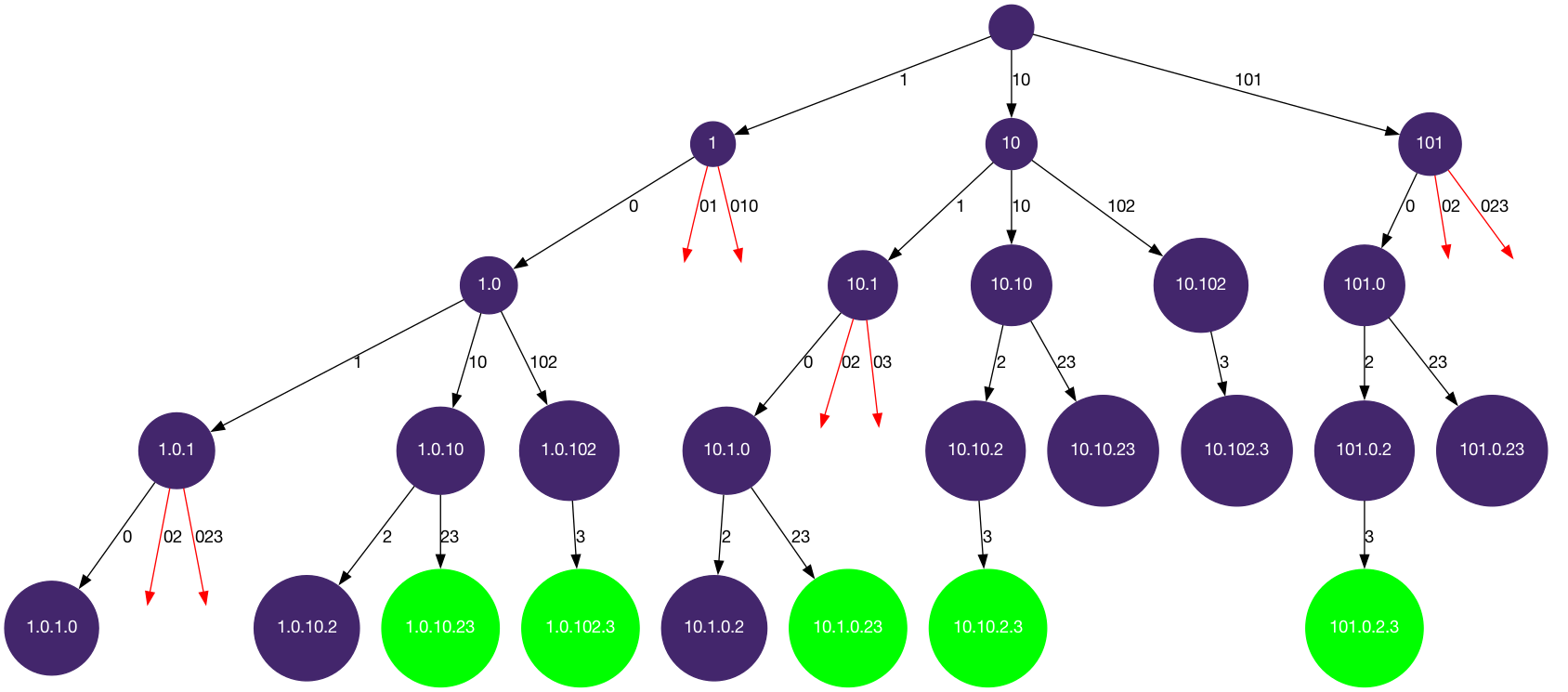# LeetCode Restore IP Addresses Solution

A valid IP address consists of exactly four integers separated by single dots. Each integer is between `0` and `255` (inclusive) and cannot have leading zeros.

For example, `"0.1.2.201"` and `"192.168.1.1"` are valid IP addresses, but `"0.011.255.245"`, `"192.168.1.312"` and `"[email protected]"` are invalid IP addresses.

Given a string `s` containing only digits, return all possible valid IP addresses that can be formed by inserting dots into `s`. You are not allowed to reorder or remove any digits in `s`. You may return the valid IP addresses in any order.

Example 1:

Input: `s = "25525511135"`

Output: `["255.255.11.135","255.255.111.35"]`

Example 2:

Input: `s = "0000"`

Output: `["0.0.0.0"]`

Example 3:

Input: `s = "101023"`

Output: `["1.0.10.23","1.0.102.3","10.1.0.23","10.10.2.3","101.0.2.3"]`

Constraints:

• `1 <= s.length <= 20`
• `s` consists of digits only.

## Solution

We want to apply our template backtracking1 to this problem. To fill in the logic:

• `is_leaf`: `start_index == len(s) and `len(path) == 4`, when all of the digits are used and there are exactly 4 segments.
• `get_edges`: the edges are the potential segments (of size 1-3) that starts at `start_index`.
• `is_valid`: is the edge (integer) between 0 to 255? and does it not have a leading zero?

#### Implementation

``````1def restoreIpAddresses(self, s: str) -> List[str]:
4        for i in range(1, 4): address += "." + path[i]
6
7    def get_edges(start_index):
8        segments = []
9        for i in range(start_index, start_index + 3):
10            if i < len(s) :    # if not out of bound
11                segments.append(s[start_index:i+1])  # up to and including s[i]
12        return segments
13
14    def is_valid(num):
15        if num == "0": return True
16        elif num == "0": return False    # leading zero
17        elif int(num) > 255: return False   # out of range
18        else: return True
19
20    def dfs(start_index, path):
21        if len(path) > 4: return
22        if start_index == len(s):   # if all digits are used
23            if len(path) == 4:      # and there are exactly four segments